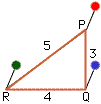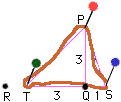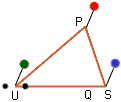Date: Tue, 3 Mar 1998 21:32:49 -0600 (CST)
Subject: Triangle area

Name: Amanda
Level: Middle

Question:
How do you figure out the area of a triangle? You already have the perimeter and height

Hi Amnanda

Knowing the perimeter and height of a triangle is not enough information to determine its area.Suppose that you have a loop of string 12 inches long stretched around three pins to form a 3-4-5 triangle as shown in the diagram. Since the angle at Q is a right angle the height of triangle PQR is 3 and its area is (1/2)base x height = 6 square inches.Now move the blue and green pins one inch to the right. You will see that the string is no longer tight. In fact since triangles PTQ and PQS are right triangles you can use the theorem of Pythagoras to find that |PT|=3 x sqrt(2) and |SP|=sqrt(10) so the perimeter of triangle PRS is 4+(3 x sqrt(2))+sqrt(10) which is approximately 11.40 inches.Remove the green pin at T and move it to the left until the string is tight, call this point U. Now triangle PUS has height 3 inches, perimeter 12 inches and its area is (1/2)x|US|x3 which is larger than 6 since |US| is larger than 4. Hence triangles PRQ and PUS have the same height and perimeter but their areas are different.
Cheers,
Penny

Go to Math Central